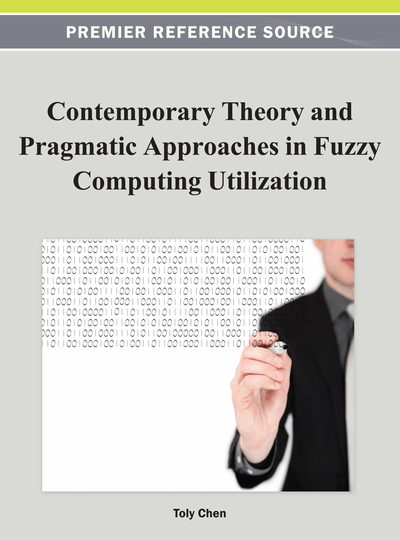# A Fuzzy Clustering Model for Fuzzy Data with Outliers

M. H. Fazel Zarandi (Amirkabir University of Technology, Iran) and Zahra S. Razaee (Amirkabir University of Technology, Iran)
DOI: 10.4018/978-1-4666-1870-1.ch003
Available
\$37.50
No Current Special Offers

## Abstract

This paper proposes a fuzzy clustering model for fuzzy data with outliers. The model is based on Wasserstein distance between interval valued data, which is generalized to fuzzy data. In addition, Keller’s approach is used to identify outliers and reduce their influences. The authors also define a transformation to change the distance to the Euclidean distance. With the help of this approach, the problem of fuzzy clustering of fuzzy data is reduced to fuzzy clustering of crisp data. In order to show the performance of the proposed clustering algorithm, two simulation experiments are discussed.
Chapter Preview
Top

## Introduction

Clustering is a division of a given set of objects into subgroups or clusters, so that objects in the same cluster are as similar as possible, and objects in different clusters are as dissimilar as possible. From a machine learning perspective, clustering is an unsupervised learning of a hidden data concept (Berkhin, 2002). In conventional (hard) clustering analysis, each datum belongs to exactly one cluster, whereas in fuzzy clustering, data points can belong to more than one cluster, and associated with each datum is a set of membership degrees. Fuzzy data are imprecise data obtained from measurements, human judgements or linguistic assessments. In cluster analysis, when there is simultaneous uncertainty in the partition and data, a fuzzy clustering model for fuzzy data should be applied (D’Urso & Giordani, 2006).

In recent literature, there are several works regarding the fuzzy clustering of fuzzy data. Hathaway et al. (1996) and Pedrycz et al. (1998) introduced models that convert parametric or non-parametric linguistic variables into generalized coordinates before performing fuzzy c-means clustering. Yang and Ko (1996) presented a fuzzy k-numbers clustering model that uses a squared distance between each pair of fuzzy numbers. Yang and Liu (1999) extended the Yang and Ko (1996) work and proposed a fuzzy k-means clustering model for conical fuzzy vectors. Yang et al. (2004) proposed a fuzzy K-means clustering model for handling both symbolic and fuzzy data. Hung and Yang (2005) proposed an alternative fuzzy k-numbers clustering model which is based on exponential-type distance measure. D’Urso and Giordani (2006) proposed a weighted fuzzy c-means clustering model which considers fuzzy data with a symmetric LR membership function.

In this paper, we first propose a new distance measure for comparison of fuzzy data. On account of the fact that all the α-cuts of fuzzy data are intervals, we obtain the distance between two fuzzy data from the distances between their α-cuts. To this purpose, a special case of Wasserstein distance is utilized. The choice of α-cuts is motivated by the fact that, fuzzy data with different shapes can be used. After introducing our distance, we use it for fuzzy clustering of fuzzy data. Moreover, with the help of Keller’s (2000) approach, an additional weighting factor is added for each datum to identify outliers and reduce their effects. In other approach, by definition of a transformation, triangular fuzzy data are changed to crisp data. With this novel approach, after applying the transformation, any fuzzy clustering model for crisp data can be used. Furthermore, for determining the optimal number of clusters, there is no need to define a cluster validity index for fuzzy data. The ones existing in literature for crisp data can be applied.

The rest of the paper is organized as follows. First, the concept of LR-type fuzzy data is introduced. Some related works regarding metrics for fuzzy data are then reviewed. We propose a distance measure for ; L(1)= 0 or (L(x) > 0,and) (Zimmerman, 2001). Then, a fuzzy number Ã is of LR-type if for c, l > 0; r > 0 in R,

(1) where, c, l, r are the center, left and right spreads of Ã, respectively. Symbolically we can write= (c, l, r).

In LR-type fuzzy numbers, the triangular fuzzy numbers (TFNs) are most commonly used. An LR-type fuzzy number Ã is called triangular fuzzy number if L(x) = R(x) = 1 | x, characterized by the following membership function:

(2) Top

In the recent literature, there are some distance measures for fuzzy data. We review some of them in this section.

## Complete Chapter List

Search this Book:
Reset